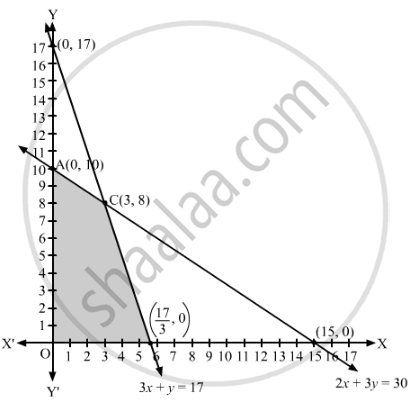# A Manufacturer Considers that Men and Women Workers Are Equally Efficient and So He Pays Them at the Same Rate. He Has 30 and 17 Units of Workers (Male and Female) and Capital Respectively, - Mathematics

Sum

A manufacturer considers that men and women workers are equally efficient and so he pays them at the same rate. He has 30 and 17 units of workers (male and female) and capital respectively, which he uses to produce two types of goods A and B. To produce one unit of A, 2 workers and 3 units of capital are required while 3 workers and 1 unit of capital is required to produce one unit of B. If A and B are priced at ₹100 and ₹120 per unit respectively, how should he use his resources to maximise the total revenue? Form the above as an LPP and solve graphically. Do you agree with this view of the manufacturer that men and women workers are equally efficient and so should be paid at the same rate?

#### Solution

Let x units of A and y units of B be produced by the manufacturer.
The price of one unit of A is ₹100 and the price of one unit of B is ₹120. Therefore, the total price of x units of A and y units of B or the total revenue is ₹(100x + 120y).

One unit of A requires 2 workers and one unit of B requires 3 workers. Therefore, xunits of A and y units of B requires (2x + 3y) workers. But, the manufacturer has 30 workers.
∴ 2x + 3≤ 30

Similarly, one unit of A requires 3 units of capital and one unit of B requires 1 unit of capital. Therefore, x units of A and y units of B requires (3x + y) units of capital. But, the manufacturer has 17 units of capital.
∴ 3x + ≤ 17
Thus, the given linear programming problem is
Maximise Z = 100+ 120y
subject to the constraints
2x + 3≤ 30
3x + ≤ 17
x, y ≥ 0
The feasible region determined by the given constraints can be diagrammatically represented as,The coordinates of the corner points of the feasible region are O(0, 0), A(0, 10), B $\left( \frac{17}{3}, 0 \right)$  and C(3, 8).

The value of the objective function at these points are given in the following table.

 Corner Point Z = 100x + 120y (0, 0) 100 × 0 + 120 × 0 = 0 (0, 10) 100 × 0 + 120 × 10 = 1200 $\left( \frac{17}{3}, 0 \right)$ 100 ×$\frac{17}{3}$ + 120 × 0 = $\frac{1700}{3}$ (3, 8) 100 × 3 + 120 × 8 = 1260   → Maximum
The maximum value of Z is 1260 at x = 3, y = 8.

Hence, the maximum total revenue is ₹1,260 when 3 units of A and 8 units of B are produced.

Yes, because the efficiency of a worker does not depend on whether the worker is a male or a female.
Concept: Graphical Method of Solving Linear Programming Problems
Is there an error in this question or solution?

#### APPEARS IN

RD Sharma Class 12 Maths
Chapter 30 Linear programming
Exercise 30.4 | Q 52 | Page 57